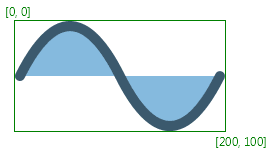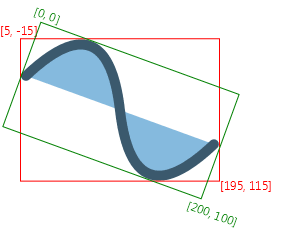@IDProperty("id")
public abstract class Node
 Comment Returned-by Constructor-argument Method-argument Field-type Type-bound Links

Base class for scene graph nodes. A scene graph is a set of tree data structures where every item has zero or one parent, and each item is either a "leaf" with zero sub-items or a "branch" with zero or more sub-items.

Each item in the scene graph is called a ` Node`. Branch nodes are of type `Parent`, whose concrete subclasses are `Group`, `javafx.scene.layout.Region`, and `javafx.scene.control.Control`, or subclasses thereof.

Leaf nodes are classes such as `javafx.scene.shape.Rectangle`, `javafx.scene.text.Text`, `javafx.scene.image.ImageView`, `javafx.scene.media.MediaView`, or other such leaf classes which cannot have children. Only a single node within each scene graph tree will have no parent, which is referred to as the "root" node.

There may be several trees in the scene graph. Some trees may be part of a `Scene`, in which case they are eligible to be displayed. Other trees might not be part of any `Scene`.

A node may occur at most once anywhere in the scene graph. Specifically, a node must appear no more than once in all of the following: as the root node of a `Scene`, the children ObservableList of a `Parent`, or as the clip of a `Node`.

The scene graph must not have cycles. A cycle would exist if a node is an ancestor of itself in the tree, considering the `Group` content ObservableList, `Parent` children ObservableList, and `Node` clip relationships mentioned above.

If a program adds a child node to a Parent (including Group, Region, etc) and that node is already a child of a different Parent or the root of a Scene, the node is automatically (and silently) removed from its former parent. If a program attempts to modify the scene graph in any other way that violates the above rules, an exception is thrown, the modification attempt is ignored and the scene graph is restored to its previous state.

It is possible to rearrange the structure of the scene graph, for example, to move a subtree from one location in the scene graph to another. In order to do this, one would normally remove the subtree from its old location before inserting it at the new location. However, the subtree will be automatically removed as described above if the application doesn't explicitly remove it.

Node objects may be constructed and modified on any thread as long they are not yet attached to a ` Scene` in a ` Window` that is showing. An application must attach nodes to such a Scene or modify them on the JavaFX Application Thread.

#### String ID

Each node in the scene graph can be given a unique `id`. This id is much like the "id" attribute of an HTML tag in that it is up to the designer and developer to ensure that the ` id` is unique within the scene graph. A convenience function called `lookup(String)` can be used to find a node with a unique id within the scene graph, or within a subtree of the scene graph. The id can also be used identify nodes for applying styles; see the CSS section below.

#### Coordinate System

The ` Node` class defines a traditional computer graphics "local" coordinate system in which the ` x` axis increases to the right and the ` y` axis increases downwards. The concrete node classes for shapes provide variables for defining the geometry and location of the shape within this local coordinate space. For example, `javafx.scene.shape.Rectangle` provides ` x`, ` y`, ` width`, ` height` variables while `javafx.scene.shape.Circle` provides ` centerX`, ` centerY`, and ` radius`.

At the device pixel level, integer coordinates map onto the corners and cracks between the pixels and the centers of the pixels appear at the midpoints between integer pixel locations. Because all coordinate values are specified with floating point numbers, coordinates can precisely point to these corners (when the floating point values have exact integer values) or to any location on the pixel. For example, a coordinate of ` (0.5, 0.5)` would point to the center of the upper left pixel on the ` Stage`. Similarly, a rectangle at ` (0, 0)` with dimensions of ` 10` by ` 10` would span from the upper left corner of the upper left pixel on the ` Stage` to the lower right corner of the 10th pixel on the 10th scanline. The pixel center of the last pixel inside that rectangle would be at the coordinates ` (9.5, 9.5)`.

In practice, most nodes have transformations applied to their coordinate system as mentioned below. As a result, the information above describing the alignment of device coordinates to the pixel grid is relative to the transformed coordinates, not the local coordinates of the nodes. The `Shape` class describes some additional important context-specific information about coordinate mapping and how it can affect rendering.

#### Transformations

Any ` Node` can have transformations applied to it. These include translation, rotation, scaling, or shearing.

A translation transformation is one which shifts the origin of the node's coordinate space along either the x or y axis. For example, if you create a `javafx.scene.shape.Rectangle` which is drawn at the origin (x=0, y=0) and has a width of 100 and a height of 50, and then apply a `javafx.scene.transform.Translate` with a shift of 10 along the x axis (x=10), then the rectangle will appear drawn at (x=10, y=0) and remain 100 points wide and 50 tall. Note that the origin was shifted, not the ` x` variable of the rectangle.

A common node transform is a translation by an integer distance, most often used to lay out nodes on the stage. Such integer translations maintain the device pixel mapping so that local coordinates that are integers still map to the cracks between pixels.

A rotation transformation is one which rotates the coordinate space of the node about a specified "pivot" point, causing the node to appear rotated. For example, if you create a `javafx.scene.shape.Rectangle` which is drawn at the origin (x=0, y=0) and has a width of 100 and height of 30 and you apply a `javafx.scene.transform.Rotate` with a 90 degree rotation (angle=90) and a pivot at the origin (pivotX=0, pivotY=0), then the rectangle will be drawn as if its x and y were zero but its height was 100 and its width -30. That is, it is as if a pin is being stuck at the top left corner and the rectangle is rotating 90 degrees clockwise around that pin. If the pivot point is instead placed in the center of the rectangle (at point x=50, y=15) then the rectangle will instead appear to rotate about its center.

Note that as with all transformations, the x, y, width, and height variables of the rectangle (which remain relative to the local coordinate space) have not changed, but rather the transformation alters the entire coordinate space of the rectangle.

A scaling transformation causes a node to either appear larger or smaller depending on the scaling factor. Scaling alters the coordinate space of the node such that each unit of distance along the axis in local coordinates is multipled by the scale factor. As with rotation transformations, scaling transformations are applied about a "pivot" point. You can think of this as the point in the Node around which you "zoom". For example, if you create a `javafx.scene.shape.Rectangle` with a ` strokeWidth` of 5, and a width and height of 50, and you apply a `javafx.scene.transform.Scale` with scale factors (x=2.0, y=2.0) and a pivot at the origin (pivotX=0, pivotY=0), the entire rectangle (including the stroke) will double in size, growing to the right and downwards from the origin.

A shearing transformation, sometimes called a skew, effectively rotates one axis so that the x and y axes are no longer perpendicular.

Multiple transformations may be applied to a node by specifying an ordered chain of transforms. The order in which the transforms are applied is defined by the ObservableList specified in the `transforms` variable.

#### Bounding Rectangles

Since every ` Node` has transformations, every Node's geometric bounding rectangle can be described differently depending on whether transformations are accounted for or not.

Each ` Node` has a read-only `boundsInLocal` variable which specifies the bounding rectangle of the ` Node` in untransformed local coordinates. ` boundsInLocal` includes the Node's shape geometry, including any space required for a non-zero stroke that may fall outside the local position/size variables, and its `clip` and `effect` variables.

Each ` Node` also has a read-only `boundsInParent` variable which specifies the bounding rectangle of the ` Node` after all transformations have been applied, including those set in `transforms`, `scaleX`/`scaleY`, `rotate`, `translateX`/`translateY`, and `layoutX`/`layoutY`. It is called "boundsInParent" because the rectangle will be relative to the parent's coordinate system. This is the 'visual' bounds of the node.

Finally, the `layoutBounds` variable defines the rectangular bounds of the ` Node` that should be used as the basis for layout calculations and may differ from the visual bounds of the node. For shapes, Text, and ImageView, layoutBounds by default includes only the shape geometry, including space required for a non-zero ` strokeWidth`, but does not include the effect, clip, or any transforms. For resizable classes (Regions and Controls) layoutBounds will always map to ` 0,0 width x height`.

The image shows a node without any transformation and its ` boundsInLocal`:If we rotate the image by 20 degrees we get following result:The red rectangle represents ` boundsInParent` in the coordinate space of the Node's parent. The ` boundsInLocal` stays the same as in the first image, the green rectangle in this image represents ` boundsInLocal` in the coordinate space of the Node.

The images show a filled and stroked rectangle and their bounds. The first rectangle ` [x:10.0 y:10.0 width:100.0 height:100.0 strokeWidth:0]` has the following bounds bounds: ` [x:10.0 y:10.0 width:100.0 height:100.0]`. The second rectangle ` [x:10.0 y:10.0 width:100.0 height:100.0 strokeWidth:5]` has the following bounds: ` [x:7.5 y:7.5 width:105 height:105]` (the stroke is centered by default, so only half of it is outside of the original bounds; it is also possible to create inside or outside stroke). Since neither of the rectangles has any transformation applied, ` boundsInParent` and ` boundsInLocal` are the same.#### CSS

The ` Node` class contains ` id`, ` styleClass`, and ` style` variables that are used in styling this node from CSS. The ` id` and ` styleClass` variables are used in CSS style sheets to identify nodes to which styles should be applied. The ` style` variable contains style properties and values that are applied directly to this node.

For further information about CSS and how to apply CSS styles to nodes, see the CSS Reference Guide.

implements EventTarget, Styleable

Since:  JavaFX 2.0